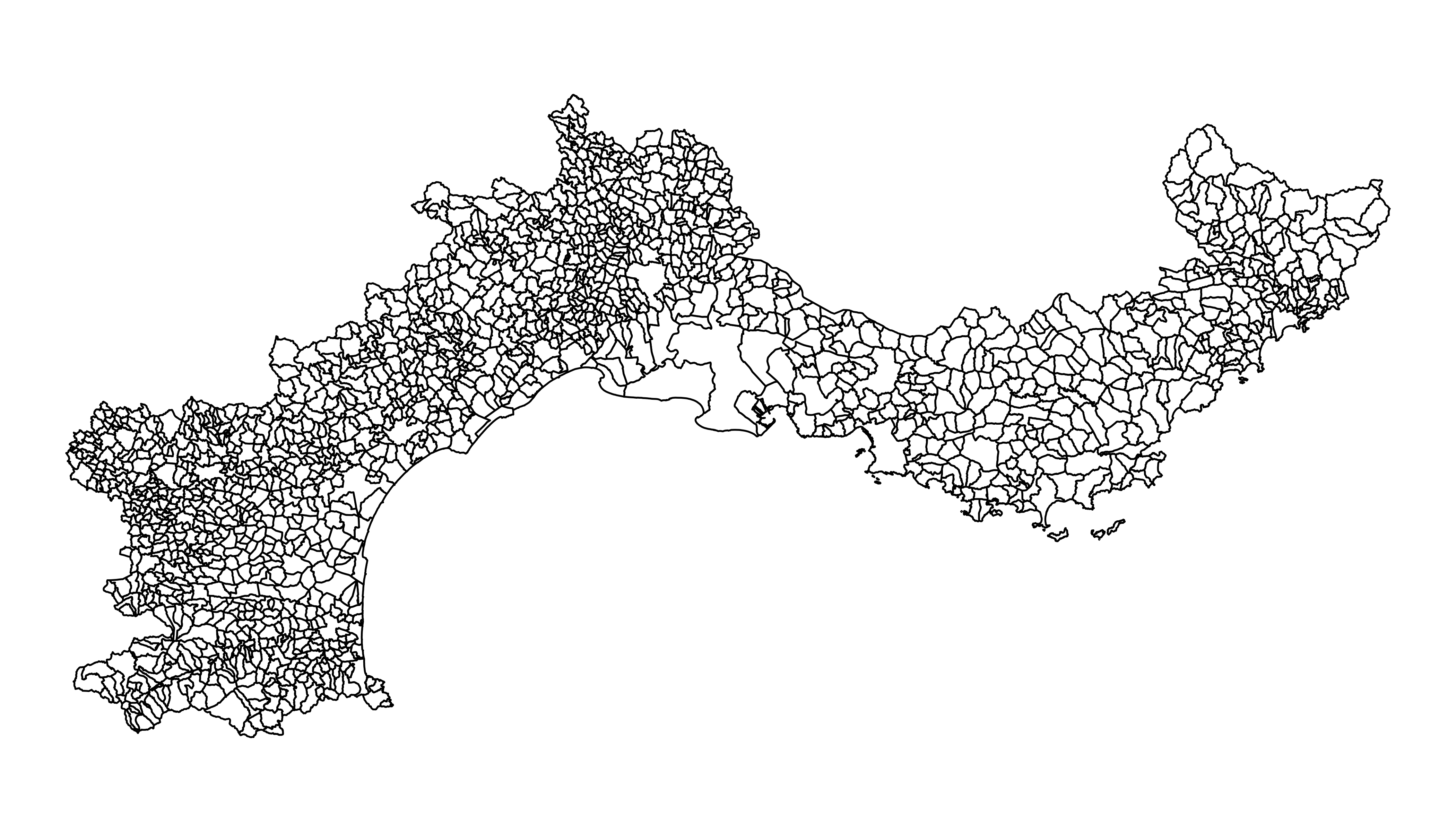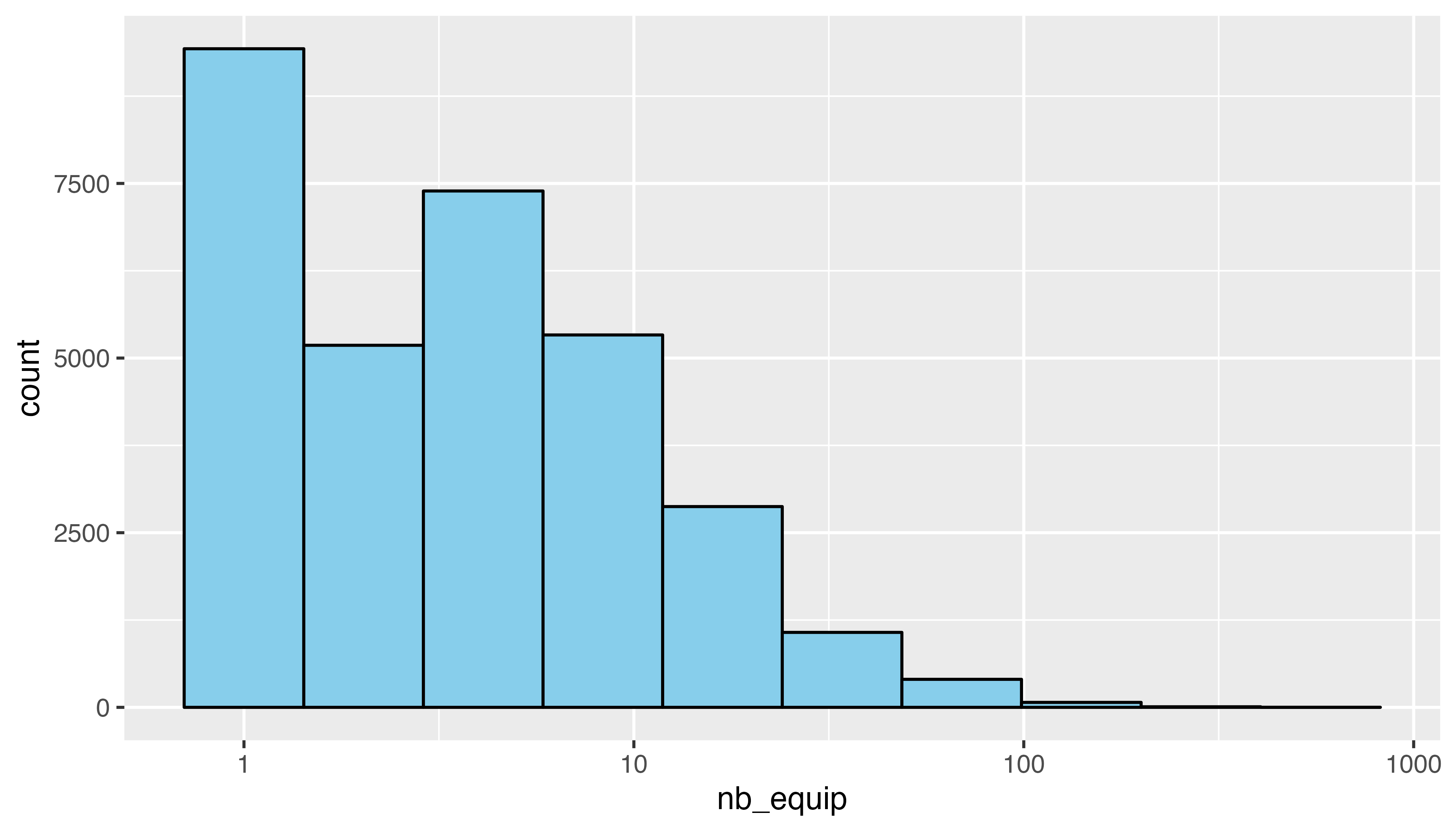# Choropleth map with R and ggplot2

This post describes how to build a choropleth map with `R` and the `ggplot2` package. It shows how to load geospatial data in R, merge region features and build the map. Reproducible code is provided.

Two inputs are needed to build a choropleth map:

• A geospatial object providing region boundaries (city districts of the south of France in this example). Data are available at the geoJSON format here, and this post explains in detail how to read and represent geoJSON format with R.
• A numeric variable that we use to color each geographical unit. Here we will use the number of restaurant per city. The data has been found here. (Thanks [@joelgombin](https://twitter.com/joelgombin)).

# Find and download a `.geoJSON` file

This step has been extensively describe in chart #325. The geojsonio library allows to read this type of format in R. To plot it with ggplot2, we first need to transform it to a data frame using the tidy function of the broom library. Then, the geom_polygon() function allows to represent this type of object !

``````# Geospatial data available at the geojson format
library(geojsonio)
spdf <- geojson_read("https://raw.githubusercontent.com/gregoiredavid/france-geojson/master/communes.geojson",  what = "sp")

# Since it is a bit too much data, I select only a subset of it:
spdf <- spdf[ substr(spdf@data\$code,1,2)  %in% c("06", "83", "13", "30", "34", "11", "66") , ]``````
``````# Geospatial data available at the geojson format
library(geojsonio)
spdf <- geojson_read("https://raw.githubusercontent.com/gregoiredavid/france-geojson/master/communes.geojson",  what = "sp")

# Since it is a bit too much data, I select only a subset of it:
spdf <- spdf[ substr(spdf@data\$code,1,2)  %in% c("06", "83", "13", "30", "34", "11", "66") , ]``````

# Basic background map

We now have a geospatial object called `spdf`. This object could be plotted as is using the `plot()` function as explained here.

However, an additionnal step is required to plot it with `ggplot2` that expects a data frame as input. It is possible to make the convertion using the `tidy` function of the `broom` package as shown below.

Finally, `geom_polygon` is used to plot the shape.``````#plot(spdf)

# I need to fortify the data AND keep trace of the commune code! (Takes ~2 minutes)
library(broom)
spdf_fortified <- tidy(spdf, region = "code")

# Now I can plot this shape easily as described before:
library(ggplot2)
ggplot() +
geom_polygon(data = spdf_fortified, aes( x = long, y = lat, group = group), fill="white", color="grey") +
theme_void() +
coord_map()``````

The number of restaurant per city district has been found on the internet and a clean version is stored on the gallery website. It is thus easy to read it with read.table. Before doing a choropleth map, it is a good practice to check the distribution of your variable.

Here, we have a ‘long tail’ distribution: a few cities have a lot of restaurant. Thus we will probably need to apply a log scale to our color palette. It will avoid that all the variation is absorbed by these high values.``````# read data

# Distribution of the number of restaurant?
library(dplyr)
data %>%
ggplot( aes(x=nb_equip)) +
geom_histogram(bins=20, fill='skyblue', color='#69b3a2') + scale_x_log10()``````

# Merge geospatial and numeric data

This is a key step in choropleth map: your 2 inputs must have a id in common to make the link between them!

``````# Make the merge
spdf_fortified = spdf_fortified %>%
left_join(. , data, by=c("id"="depcom"))

# Note that if the number of restaurant is NA, it is in fact 0
spdf_fortified\$nb_equip[ is.na(spdf_fortified\$nb_equip)] = 0.001``````

We can make a first basic choropleth map. We just need to add fill = our value in the aesthetic of our polygons.``````ggplot() +
geom_polygon(data = spdf_fortified, aes(fill = nb_equip, x = long, y = lat, group = group)) +
theme_void() +
coord_map()``````

# Customized choropleth map with R and ggplot2

There is a bit of work to do to get a descent figure. We need to change the color palette, improve the legend, use a log scale transformation for the colorscale, change background and add titles and explanation. Here is the code to do that, and the final result!

``````library(viridis)
p <- ggplot() +
geom_polygon(data = spdf_fortified, aes(fill = nb_equip, x = long, y = lat, group = group) , size=0, alpha=0.9) +
theme_void() +
scale_fill_viridis(trans = "log", breaks=c(1,5,10,20,50,100), name="Number of restaurant", guide = guide_legend( keyheight = unit(3, units = "mm"), keywidth=unit(12, units = "mm"), label.position = "bottom", title.position = 'top', nrow=1) ) +
labs(
title = "South of France Restaurant concentration",
subtitle = "Number of restaurant per city district",
caption = "Data: INSEE | Creation: Yan Holtz | r-graph-gallery.com"
) +
theme(
text = element_text(color = "#22211d"),
plot.background = element_rect(fill = "#f5f5f2", color = NA),
panel.background = element_rect(fill = "#f5f5f2", color = NA),
legend.background = element_rect(fill = "#f5f5f2", color = NA),

plot.title = element_text(size= 22, hjust=0.01, color = "#4e4d47", margin = margin(b = -0.1, t = 0.4, l = 2, unit = "cm")),
plot.subtitle = element_text(size= 17, hjust=0.01, color = "#4e4d47", margin = margin(b = -0.1, t = 0.43, l = 2, unit = "cm")),
plot.caption = element_text( size=12, color = "#4e4d47", margin = margin(b = 0.3, r=-99, unit = "cm") ),

legend.position = c(0.7, 0.09)
) +
coord_map()
p``````Related chart types

## Contact

This document is a work by Yan Holtz. Any feedback is highly encouraged. You can fill an issue on Github, drop me a message on Twitter, or send an email pasting yan.holtz.data with gmail.com.